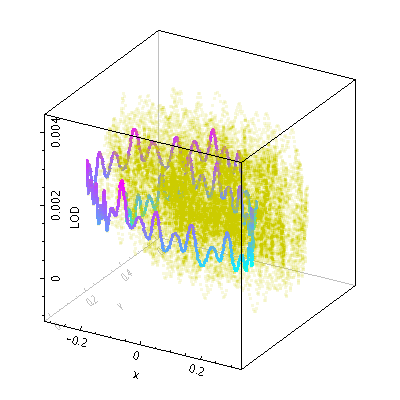Next Previous Up Contents
Next: spheregrid
Up: Layer Types
Previous: xyzerror

#### 8.3.43 `line3d`

Plots a point-to-point line joining up the positions of data points in three dimensions. There are additional options to pre-sort the points by a given quantity before drawing the lines (using the `sort` value), and to vary the colour of the line along its length (using the `aux` value). The options for controlling the Aux colour map are controlled at the level of the plot itself, rather than by per-layer configuration.

Note that the line positioning in 3d and the line segment aux colouring is somewhat approximate. In most cases it is good enough for visual inspection, but pixel-level examination may reveal discrepancies.

Usage Overview:

```   layerN=line3d colorN=<rrggbb>|red|blue|... thickN=<pixels>
<pos-coord-paramsN> auxN=<num-expr> sortN=<num-expr>
inN=<table> ifmtN=<in-format> istreamN=true|false icmdN=<cmds>
```

All the parameters listed here affect only the relevant layer, identified by the suffix `N`.

Positional Coordinate Parameters:
The positional coordinates `<pos-coord-paramsN>` give a position for each row of the input table. Their form depends on the plot geometry, i.e. which plotting command is used. For a plane plot (`plot2plane`) the parameters would be `xN` and `yN`. The coordinate parameter values are in all cases strings interpreted as numeric expressions based on column names. These can be column names, fixed values or algebraic expressions as described in Section 10.

Example:```   stilts plot2cube in=iers.fits x=x y=y z=LOD
layer1=line3d icmd1='select decYear>1963&&decYear<1964.5' thick1=3 aux1=LOD
auxmap=cyan-magenta auxvisible=false legend=false
phi=-150 theta=25 psi=180 xpix=400 ypix=400```

`auxN = <num-expr>`       (String)
If supplied, this adjusts the colouring of the line along its length according to the value of this coordinate.

The value is a numeric algebraic expression based on column names as described in Section 10.

`colorN = <rrggbb>|red|blue|...`       (Color)
The color of plotted data, given by name or as a hexadecimal RGB value.

The standard plotting colour names are `red`, `blue`, `green`, `grey`, `magenta`, `cyan`, `orange`, `pink`, `yellow`, `black`, `light_grey`, `white`. However, many other common colour names (too many to list here) are also understood. The list currently contains those colour names understood by most web browsers, from `AliceBlue` to `YellowGreen`, listed e.g. in the Extended color keywords section of the CSS3 standard.

Alternatively, a six-digit hexadecimal number RRGGBB may be supplied, optionally prefixed by "`#`" or "`0x`", giving red, green and blue intensities, e.g. "`ff00ff`", "`#ff00ff`" or "`0xff00ff`" for magenta.

[Default: `red`]

`icmdN = <cmds>`       (ProcessingStep[])
Specifies processing to be performed on the layer N input table as specified by parameter `inN`. The value of this parameter is one or more of the filter commands described in Section 6.1. If more than one is given, they must be separated by semicolon characters (";"). This parameter can be repeated multiple times on the same command line to build up a list of processing steps. The sequence of commands given in this way defines the processing pipeline which is performed on the table.

Commands may alteratively be supplied in an external file, by using the indirection character '@'. Thus a value of "`@filename`" causes the file `filename` to be read for a list of filter commands to execute. The commands in the file may be separated by newline characters and/or semicolons, and lines which are blank or which start with a '`#`' character are ignored.

`ifmtN = <in-format>`       (String)
Specifies the format of the input table as specified by parameter `inN`. The known formats are listed in Section 5.1.1. This flag can be used if you know what format your table is in. If it has the special value `(auto)` (the default), then an attempt will be made to detect the format of the table automatically. This cannot always be done correctly however, in which case the program will exit with an error explaining which formats were attempted. This parameter is ignored for scheme-specified tables.

[Default: `(auto)`]

`inN = <table>`       (StarTable)
The location of the input table. This may take one of the following forms:
• A filename.
• A URL.
• The special value "`-`", meaning standard input. In this case the input format must be given explicitly using the `ifmtN` parameter. Note that not all formats can be streamed in this way.
• A scheme specification of the form `:<scheme-name>:<scheme-args>`.
• A system command line with either a "`<`" character at the start, or a "`|`" character at the end ("`<syscmd`" or "`syscmd|`"). This executes the given pipeline and reads from its standard output. This will probably only work on unix-like systems.
In any case, compressed data in one of the supported compression formats (gzip, Unix compress or bzip2) will be decompressed transparently.
`istreamN = true|false`       (Boolean)
If set true, the input table specified by the `inN` parameter will be read as a stream. It is necessary to give the `ifmtN` parameter in this case. Depending on the required operations and processing mode, this may cause the read to fail (sometimes it is necessary to read the table more than once). It is not normally necessary to set this flag; in most cases the data will be streamed automatically if that is the best thing to do. However it can sometimes result in less resource usage when processing large files in certain formats (such as VOTable). This parameter is ignored for scheme-specified tables.

[Default: `false`]

`sortN = <num-expr>`       (String)
If supplied, this gives a value to define in what order points are joined together. If no value is given, the natural order is used, i.e. the sequence of rows in the table.

Note that if the required order is in fact the natural order of the table, it is better to leave this value blank, since sorting is a potentially expensive step.

The value is a numeric algebraic expression based on column names as described in Section 10.

`thickN = <pixels>`       (Integer)
Thickness of plotted line in pixels.

[Default: `1`]

Next Previous Up Contents
Next: spheregrid
Up: Layer Types
Previous: xyzerror

STILTS - Starlink Tables Infrastructure Library Tool Set